# Physics Homework Help With Linear Momentum

## Linear Momentum

The momentum of a single particle is a vector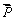defined as the product of its mass and its velocity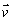. That is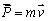Suppose that instead of a single particle we have a system of n particle with masses m1, m2 … etc, and their velocities v1 and v2 etc. respectively then the total momentum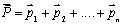in a particular reference frame is,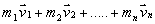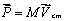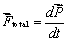The vector sum of all external forces is given as,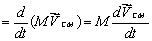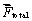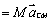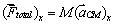Taking its components along x-direction and y-direction we can write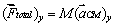This is nothing but Newton's second laws of motion. Note that no internal force will be used in this expression and only external forces will be used.

Conservation of Linear Momentum

If the sum of the external force acting on a system is zero. Then,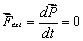or= constant.

So, when the resultant external force acting on the system is zero, the total vector momentum of the system remains constant. This is called the principle of the conservation of linear momentum. The momentum of the individual particles may change, but their sum remains constant if there is no net external force.

### Homework Help For Linear Momentum

assignmenthelp.net provides best Online Assignment Help service in Physics for all standards. Our Tutor provide their high quality and optimized Tutorial help to fulfill all kind of need of Students.